# Digital Electronics - Boolean Algebra and Logic Simplification

### Exercise :: Boolean Algebra and Logic Simplification - Filling the Blanks

1.

The Boolean expression C + CD is equal to ________.

 A. C B. D C. C + D D. 1

Answer: Option A

Explanation:

No answer description available for this question. Let us discuss.

2.

The Boolean expression for the logic circuit shown is _____.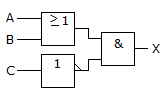A.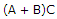B.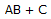C.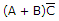D.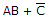Answer: Option C

Explanation:

No answer description available for this question. Let us discuss.

3.

Applying DeMorgan's theorem and Boolean algebra to the expression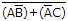results in ________.

 A.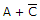B.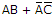C.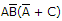D.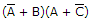Answer: Option D

Explanation:

No answer description available for this question. Let us discuss.

4.

The standard SOP form of the expression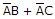is ________.

 A.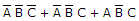B.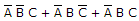C.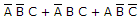D.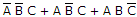Answer: Option B

Explanation:

No answer description available for this question. Let us discuss.

5.

Identify the Boolean expression that is in standard POS form.

 A.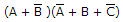B.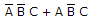C.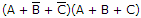D. (A + B)(C + D)

Answer: Option C

Explanation:

No answer description available for this question. Let us discuss.

#### Current Affairs 2021

Interview Questions and Answers